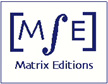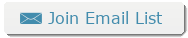Matrix Editions
serious mathematics,
written with the reader in mind

# Future Books

## Functional Analysis Volume 2

### The spectral theorem for normal compact operators

#### Dzung Minh Ha

No publication date has been set; we are still awaiting files from Professor Ha.

### Tentative list of topics

Measure and integration
Normed and topological vector spaces; Hilbert spaces
Schauder bases
coefficient functionals and basic sequences equivalent bases
Schauder basis for C([a,b], R)
The Hahn-Banach theorem and its consequences
Separation theorems
Dual spaces of some well-known spaces
Dual spaces of closed subspaces and quotient spaces
Basic sequences in dual spaces
The bi-dual and reflexivity
Characterizations of reflexivity
Weak and weak star toplogy
Tukey's lemma and Tychonov's theorem on product of compact topological spaces
Weak convergence in lp
Weak completeness of reflexive normed spaces
Schur's theorem on the equivalence of weak and strong convergence in l1
A Bolzano-Weierstrass theorem for weak convergence
The Banach-Alaoglu theorem
Operators on Hilbert spaces
Spectral theory of Banach algebras
The spectral radius formula and the spectral mapping theorem
Gelfand-Mazur theorem and Wienner's theorem
Compact operators and finite-rank operators
spectral theory of compact operators
The spectral theorem for compact normal operators on Hilbert space

Would you like to be notified when this book is available?